### Through my heart, through my soul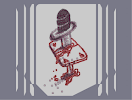Hover over the thumbnail for a full-size version.

Author Dragon_Moon author:dragon_moon bitesized n-art rated 2007-05-31 2007-11-01 4 by 85 people. \$Through my heart, through my soul#Dragon_Moon#none#1111111111111111111111111111111111111111111111111111111111111111111111LOOOOOOOOOOOOOKLOOOOK111111111111111111111111LOOOOOOOOOOOOOOOOKLOOOKMQQQQQQQQQQQQQQQQQQJQQJOOOOOOOOOOOOOOOOOOOOLOO9000000000000000000021100000000000000000000021000000000000000000000020000000000000000000000000000000000000000000000000000000000000000000000000000000000000000000000000000000000000000000000000000000000000000000000000000000000000000?0000000000000000000000C000000000000000000000?1000000000000000000000C100000000000000000000?1100000000000000000000C11QQQQQQQQQQQQQQQQQQQQMQQLOOOOOOOOOOOOOOOOOOKOOKMQQQQQQQQQQQQQQQQJMQQQJ111111111111111111111111MQQQQQQQQQQQQQQJMQQQJ1111111111111111111111111111111111111111111111111111111111111111111111|10^377,58!10^374,59!10^372,62!10^371,63!10^368,65!10^366,65!10^366,65!10^362,68!10^358,72!10^357,75!10^355,78!10^353,80!10^352,83!10^350,87!10^348,90!10^347,94!10^347,98!10^347,101!10^347,104!10^346,105!10^346,108!10^349,109!10^354,109!10^357,109!10^361,109!10^364,107!10^366,105!10^369,105!10^374,103!10^379,102!10^384,100!10^387,98!10^389,97!10^391,93!10^393,92!10^395,88!10^399,85!10^401,82!10^403,78!10^405,75!10^404,72!10^402,69!10^399,67!10^397,67!10^394,65!10^392,64!10^388,64!10^386,63!10^383,63!10^380,63!10^379,63!10^377,63!10^400,68!10^396,64!10^392,62!10^390,62!10^386,60!10^385,60!10^383,59!10^383,59!10^345,126!10^348,126!10^348,126!10^351,126!10^353,126!10^356,126!10^359,125!10^361,124!10^363,123!10^366,123!10^368,123!10^375,122!10^379,122!10^383,120!10^386,118!10^389,117!10^391,115!10^392,113!10^394,112!10^396,108!10^399,105!10^401,104!10^403,103!10^404,102!10^406,100!10^407,97!10^409,95!10^410,94!10^411,92!10^411,87!10^411,81!10^411,81!10^408,77!10^408,77!10^413,83!10^413,87!10^345,128!10^345,128!10^345,130!10^346,134!10^346,139!10^347,141!10^348,144!10^349,147!10^351,147!10^356,148!10^358,148!10^362,147!10^367,147!10^372,145!10^374,144!10^377,143!10^381,141!10^385,140!10^392,136!10^394,135!10^397,133!10^399,132!10^402,130!10^404,129!10^406,127!10^411,123!10^412,121!10^414,118!10^416,113!10^416,110!10^416,108!10^416,105!10^416,100!10^416,96!10^416,93!10^416,90!10^354,169!10^355,169!10^359,168!10^364,167!10^366,165!10^368,165!10^369,165!10^373,163!10^375,163!10^378,162!10^379,161!10^382,160!10^384,158!10^386,158!10^388,156!10^391,155!10^394,154!10^396,153!10^398,152!10^401,150!10^404,148!10^408,147!10^411,144!10^411,142!10^413,140!10^415,137!10^416,135!10^416,133!10^417,130!10^417,127!10^417,125!10^418,124!10^353,170!10^354,171!10^355,175!10^356,176!10^356,178!10^358,180!10^358,181!10^353,177!10^353,183!10^353,184!10^354,188!10^354,191!10^356,192!10^356,193!10^359,193!10^361,193!10^363,193!10^364,193!10^368,192!10^371,190!10^373,188!10^375,187!10^378,185!10^381,184!10^386,182!10^389,180!10^393,179!10^394,178!10^396,177!10^379,185!10^391,183!10^396,180!10^396,178!10^399,177!10^401,175!10^403,173!10^405,171!10^408,170!10^410,167!10^411,166!10^413,165!10^414,163!10^415,161!10^416,157!10^416,148!10^416,148!10^417,142!10^418,138!10^418,133!10^418,128!10^417,124!10^417,122!10^418,118!10^417,113!10^415,159!10^418,150!10^418,147!10^418,135!10^351,168!10^350,167!10^350,165!10^350,165!10^350,168!10^351,173!10^350,180!10^338,233!10^337,233!10^333,234!10^330,234!10^328,234!10^323,234!10^318,233!10^315,232!10^315,232!10^310,229!10^310,228!10^307,225!10^307,222!10^306,221!10^305,219!10^305,216!10^305,214!10^305,211!10^305,211!10^305,216!10^305,219!10^308,228!10^314,234!10^321,235!10^328,236!10^331,235!10^336,234!10^339,232!10^342,231!10^346,230!10^349,228!10^351,228!10^354,225!10^357,223!10^359,222!10^354,228!10^360,226!10^363,224!10^364,223!10^368,220!10^428,137!10^429,137!10^431,137!10^436,137!10^439,139!10^441,141!10^444,144!10^445,147!10^446,151!10^447,154!10^447,156!10^445,160!10^442,164!10^440,167!10^439,168!10^443,157!10^442,147!10^437,139!10^429,137!10^422,137!10^415,137!3^307,204!3^313,195!3^321,190!3^330,184!3^337,177!3^314,203!3^316,213!3^325,222!3^337,222!3^347,216!3^360,213!3^373,217!3^383,208!3^373,210!3^390,203!3^401,197!3^410,190!3^419,185!3^429,180!3^435,172!3^435,158!3^431,145!3^348,196!3^340,204!3^353,201!3^367,196!3^348,173!3^351,158!3^363,158!3^374,153!3^386,149!3^393,143!3^405,137!3^348,114!3^355,118!3^359,118!3^369,116!3^377,113!3^385,108!3^395,95!3^402,88!10^449,153!3^446,163!3^451,153!3^453,146!3^452,138!3^444,133!3^433,132!3^429,134!3^451,156!3^302,224!3^303,231!3^303,231!3^306,235!3^309,239!3^311,241!3^312,243!3^319,243!3^326,245!3^331,243!3^339,239!3^338,243!3^341,242!3^344,239!3^350,237!3^355,236!3^358,233!3^362,232!3^366,228!3^371,225!3^376,223!3^378,219!3^386,215!3^398,208!3^409,201!3^419,193!3^427,189!3^436,181!3^442,175!3^450,167!3^456,157!3^378,217!3^392,212!3^404,204!10^354,102!10^354,96!10^354,90!10^360,90!10^366,90!10^372,90!10^378,90!10^384,90!10^384,96!10^378,96!10^372,96!10^366,96!10^360,96!10^360,102!10^366,102!10^372,102!10^360,84!10^366,84!10^366,84!10^372,84!10^378,84!10^378,84!10^384,84!10^390,84!10^396,84!10^402,72!10^396,72!10^390,72!10^384,72!10^378,72!10^372,72!10^366,72!10^366,78!10^372,78!10^378,78!10^384,78!10^390,78!10^360,78!10^354,132!10^360,132!10^366,132!10^372,132!10^378,132!10^384,132!10^390,132!10^396,126!10^390,126!10^384,126!10^378,126!10^354,138!10^360,138!10^366,138!10^372,138!10^378,138!10^360,144!10^366,144!10^396,120!10^402,120!10^408,120!10^408,114!10^402,114!10^402,108!10^408,108!10^408,102!10^360,174!10^366,174!10^372,174!10^378,174!10^384,174!10^396,174!10^390,174!10^378,168!10^384,168!10^390,168!10^396,168!10^402,168!10^402,162!10^396,162!10^384,162!10^390,162!10^402,156!10^408,156!10^360,180!10^372,180!10^366,186!3^348,198!3^356,199!3^368,196!3^378,193!3^343,196!3^334,206!3^317,216!3^317,219!3^315,221!3^323,223!3^370,237!3^370,244!3^371,252!3^372,260!3^372,270!3^376,281!3^385,280!3^393,276!3^403,272!3^423,225!3^423,235!3^424,245!3^424,253!3^424,262!3^392,270!3^390,262!3^388,251!3^387,242!3^385,235!3^385,227!3^422,218!3^421,212!3^418,205!3^419,199!12^324,307!12^326,311!12^328,315!12^331,319!12^331,322!12^334,325!12^336,327!12^338,330!12^341,334!12^342,336!12^344,338!12^346,340!12^347,342!12^348,347!12^349,348!12^352,351!12^354,354!12^355,356!12^357,358!12^358,358!12^358,359!12^359,361!12^361,364!12^361,367!12^363,371!12^363,373!12^364,373!12^365,374!12^367,376!12^369,379!12^370,381!12^371,383!12^373,383!12^374,386!12^376,386!12^379,387!12^383,388!12^385,388!12^387,388!12^390,388!12^394,386!12^397,385!12^400,385!12^404,383!12^408,382!12^413,380!12^416,379!12^421,378!12^425,377!12^427,375!12^429,375!12^433,375!12^436,374!12^441,372!12^445,368!12^449,367!12^454,365!12^457,365!12^459,365!12^464,363!12^468,362!12^472,360!12^474,360!12^479,358!12^483,357!12^486,357!12^489,356!12^491,355!12^494,355!12^496,354!12^500,353!12^503,352!12^506,349!12^509,347!12^512,345!12^514,342!12^517,340!12^518,337!12^518,334!12^517,330!12^518,327!12^518,324!12^515,321!12^516,320!12^512,317!12^510,316!12^510,314!12^509,312!12^507,308!12^503,305!12^502,302!12^500,300!12^500,300!12^500,298!12^498,295!12^496,293!12^492,290!12^492,290!12^490,289!12^487,285!12^484,282!12^483,282!12^480,279!12^480,279!12^479,277!12^475,272!12^473,271!12^470,268!12^468,267!12^466,265!12^464,264!12^462,262!12^461,261!12^459,258!12^458,258!12^457,256!12^457,255!12^454,252!12^453,250!12^451,247!12^449,247!12^448,246!12^446,244!12^444,243!12^442,241!12^440,239!12^438,237!12^437,235!12^434,233!12^432,232!12^431,230!12^516,323!12^511,317!12^505,310!12^503,307!12^499,303!12^496,298!12^493,292!12^488,287!12^324,308!12^324,308!12^324,306!12^320,302!12^317,300!12^316,299!12^315,298!12^313,295!12^310,291!12^310,290!12^308,287!12^306,284!12^305,283!12^304,280!12^303,278!12^301,277!12^300,275!12^298,272!12^298,270!12^297,267!12^297,265!12^297,263!12^297,262!12^297,262!12^299,259!12^302,256!12^304,255!12^307,253!12^306,253!12^309,253!12^311,250!12^312,250!12^315,249!12^317,247!10^370,287!10^370,287!10^376,288!10^376,288!10^399,278!10^421,269!10^423,268!10^409,273!10^385,283!10^394,282!10^413,270!3^399,268!3^398,257!3^396,243!3^393,234!3^393,225!3^409,261!3^406,246!3^405,229!3^403,213!12^336,323!12^339,321!12^341,320!12^343,319!12^344,318!12^346,317!12^348,316!12^349,315!12^352,314!12^356,313!12^358,312!12^358,311!12^361,310!12^362,310!12^365,308!12^365,308!12^368,307!12^370,307!12^372,306!12^375,303!12^377,303!12^379,302!12^382,300!12^383,299!12^385,299!12^386,299!12^390,297!12^392,297!12^396,294!12^397,293!12^398,293!12^400,293!12^403,293!12^406,291!12^408,290!12^411,289!12^413,288!12^414,288!12^418,287!12^419,286!12^421,285!12^426,284!12^428,283!12^430,282!12^433,282!12^436,280!12^439,279!12^441,278!12^444,277!12^445,277!12^449,275!12^451,274!12^454,273!12^456,272!12^459,270!12^461,268!12^463,268!12^323,262!12^322,262!12^321,262!12^319,263!12^318,264!12^318,267!12^318,270!12^319,273!12^321,274!12^324,275!12^326,276!12^328,276!12^330,278!12^331,278!12^331,278!12^332,275!12^332,273!12^333,270!12^333,268!12^334,266!12^334,263!12^334,263!12^333,262!12^332,262!12^330,263!12^328,266!12^328,266!12^328,271!12^329,275!12^329,275!12^326,272!12^328,269!12^319,262!12^321,269!12^323,269!12^331,264!12^330,264!12^330,261!12^330,261!12^331,261!12^331,259!12^331,258!12^332,259!12^333,261!12^333,264!12^390,372!12^390,373!12^390,372!12^388,368!12^387,367!12^385,364!12^385,361!12^385,360!12^384,357!12^383,356!12^383,356!12^385,356!12^388,358!12^392,360!12^395,361!12^398,363!12^400,364!12^403,368!12^403,369!12^401,371!12^398,371!12^396,370!12^395,368!12^394,368!12^394,369!12^393,372!12^392,373!12^390,373!12^389,370!12^387,367!12^387,363!12^389,363!12^394,367!12^404,370!12^407,370!12^409,370!12^409,367!12^409,365!12^407,363!12^404,362!12^402,360!12^398,359!12^395,358!12^392,357!12^390,355!12^388,355!12^393,376!12^391,376!12^388,376!12^387,372!12^387,368!12^385,363!12^376,392!12^375,392!12^372,390!12^371,387!12^367,382!12^364,376!12^362,373!12^358,369!12^357,366!12^355,363!12^351,357!12^348,355!12^347,352!12^345,349!12^342,345!12^340,342!12^337,339!12^334,337!12^333,335!12^331,333!12^329,331!12^327,327!12^325,324!12^323,320!12^321,318!12^320,314!12^317,311!12^316,308!12^313,305!12^311,301!12^308,297!12^307,292!12^305,288!12^302,285!12^370,387!12^368,385!12^367,380!12^364,377!12^362,375!12^360,370!12^357,367!12^354,363!12^351,358!12^347,355!12^343,352!12^339,348!12^338,345!12^335,341!12^333,338!12^332,335!12^330,332!12^344,357!12^344,357!12^345,358!12^346,360!12^348,363!12^348,365!12^351,368!12^352,369!12^354,373!12^356,377!12^359,380!12^359,382!12^362,384!12^366,388!12^366,390!12^368,390!12^368,391!12^371,393!12^373,394!12^374,395!12^376,398!12^379,399!12^383,399!12^388,399!12^391,398!12^394,398!12^398,397!12^401,397!12^405,395!12^408,395!12^411,394!12^414,392!12^418,392!12^421,390!12^426,388!12^429,387!12^432,387!12^434,386!12^437,386!12^439,384!12^441,383!12^446,382!12^449,380!12^453,379!12^455,378!12^458,378!12^459,377!12^463,376!12^465,375!12^468,375!12^471,373!12^475,372!12^478,370!12^483,368!12^486,367!12^488,367!12^491,366!12^494,365!12^497,364!12^501,363!12^504,361!12^506,360!12^509,360!12^513,358!12^516,357!12^519,355!12^521,352!12^521,348!12^522,343!12^523,337!12^522,337!12^522,333!12^522,332!12^517,327!12^514,325!12^383,396!12^393,392!12^416,385!12^446,375!12^498,340!12^498,340!12^498,340!12^499,338!12^503,338!12^504,337!12^504,333!12^502,330!12^501,329!12^498,328!12^497,328!12^493,327!12^492,326!12^489,325!12^485,323!12^483,323!12^482,323!12^482,323!12^482,323!12^482,328!12^482,329!12^483,333!12^483,335!12^483,337!12^484,338!12^485,341!12^488,342!12^489,343!12^491,343!12^492,342!12^493,340!12^494,337!12^489,335!12^486,334!12^486,334!12^486,333!12^489,333!12^493,335!12^493,335!12^493,333!12^502,333!12^495,333!12^491,332!12^489,330!12^486,328!12^483,326!12^482,325!12^479,325!12^478,324!12^478,322!12^477,320!12^477,319!12^477,318!12^480,319!12^486,324!12^489,326!12^493,327!12^481,328!12^480,329!12^480,330!12^480,332!12^432,389!3^433,391!3^436,400!3^436,409!3^438,420!3^439,432!3^432,438!3^422,445!3^404,404!3^405,413!3^406,423!3^408,434!3^409,446!3^415,445!3^423,443!3^427,435!3^425,429!3^423,422!3^421,415!3^420,408!3^420,402!3^432,426!3^429,417!12^431,259!12^433,258!12^433,258!12^420,283!12^437,260!12^439,260!12^439,260!12^441,261!12^442,264!12^443,266!12^442,269!12^439,271!12^439,272!12^437,275!12^435,276!12^433,279!12^432,281!12^429,284!12^428,288!12^427,291!12^429,276!12^429,276!12^428,274!12^427,273!12^427,272!12^427,270!12^427,270!12^420,274!12^417,276!12^412,278!12^409,279!12^414,275!12^412,276!12^405,281!12^401,284!12^400,284!12^398,286!12^397,286!12^397,288!12^430,287!12^427,293!12^425,297!12^422,302!12^422,304!12^420,305!12^418,307!12^415,309!12^412,313!12^410,314!12^408,314!12^404,314!12^401,315!12^397,315!12^392,317!12^388,318!12^385,319!12^382,321!12^380,323!12^379,326!12^378,329!12^376,331!12^373,333!12^371,334!12^367,337!12^364,337!12^358,338!12^355,341!12^353,343!12^353,344!12^408,293!12^404,298!12^400,301!12^394,304!12^387,307!12^380,309!12^375,313!12^370,318!12^367,321!12^363,324!12^360,327!12^355,328!12^353,328!12^349,330!12^347,333!12^408,310!12^405,310!12^405,308!12^406,303!12^406,300!12^408,297!12^405,298!12^407,307!12^409,307!12^412,307!12^413,305!12^414,302!12^405,305!12^403,309!12^398,309!12^395,311!12^389,312!12^395,308!12^390,309!12^383,312!12^378,314!12^375,316!12^370,318!12^367,322!12^367,326!12^362,329!12^358,331!12^354,331!12^350,334!12^347,339!12^348,341!12^351,335!12^358,334!12^395,289!12^388,289!12^383,291!12^377,293!12^373,293!12^369,293!12^377,294!12^367,294!12^363,294!12^362,293!12^362,290!12^362,289!12^361,289!12^354,291!12^349,294!12^346,295!12^340,299!12^337,302!12^335,304!12^333,308!12^329,308!12^331,308!12^336,305!12^339,300!12^345,295!12^349,294!12^354,293!12^358,293!12^417,317!12^414,318!12^412,323!12^411,329!12^409,332!12^407,336!12^406,336!12^407,333!12^409,325!12^408,322!12^408,318!12^408,314!12^410,314!12^412,316!12^419,317!12^419,315!12^363,288!12^365,285!12^366,284!12^368,283!12^390,302!12^394,300!12^398,298!12^398,297!12^400,297!12^396,299!12^404,308!12^403,308!12^403,307!12^392,293!12^390,296!12^388,298!12^386,298!12^385,297!12^393,294!12^390,293!12^383,293!12^378,294!12^368,289!12^369,288!12^375,288!12^379,288!12^380,288!12^384,285!12^388,284!12^400,291!12^403,287!12^407,283!12^413,282!12^415,278!12^421,277!12^423,281!3^378,375!3^363,354!3^323,292!3^306,267!3^365,285!3^350,300!3^337,313!3^360,297!3^369,289!3^348,306!3^376,295!3^386,295!3^396,285!10^357,293!10^364,287!10^381,288!3^413,369!3^432,359!3^463,351!3^378,347!12^373,257!12^373,257!12^372,254!12^372,254!12^374,259!12^374,261!12^377,265!12^377,269!12^374,269!12^374,269!12^372,263!12^373,270!12^376,272!12^338,353!12^341,356!12^341,358!12^341,363!12^341,366!12^339,371!12^337,374!12^337,377!12^338,380!12^341,380!12^341,380!12^346,376!12^346,374!12^348,372!12^407,399!12^406,404!12^405,408!12^405,410!12^405,414!12^404,419!12^403,424!12^403,428!12^404,432!12^405,436!12^403,442!12^405,446!12^406,448!12^405,443!12^403,435!12^407,429!12^409,423!12^407,415!12^406,410!12^406,408!12^426,438!12^429,437!12^433,436!12^432,431!12^433,423!12^433,423!12^435,431!12^434,422!12^432,415!12^424,415!12^421,406!12^419,400!12^419,398!12^436,417!12^437,413!12^438,408!12^437,403!12^435,398!12^435,393!12^435,392!12^447,420!12^449,418!12^452,417!12^455,415!12^460,415!12^463,415!12^466,417!12^466,423!12^466,426!12^469,430!12^472,431!12^474,433!12^477,436!12^478,441!12^478,444!12^477,448!12^473,453!12^470,454!12^465,456!12^458,456!12^453,455!12^447,455!12^441,456!12^438,458!12^433,465!12^431,469!12^430,473!12^430,479!12^428,484!12^425,488!12^420,493!12^414,496!12^408,501!12^405,507!12^402,509!12^398,509!12^395,509!12^394,504!12^397,500!12^398,497!12^398,492!12^399,487!12^399,483!12^396,478!12^393,475!12^389,472!12^383,472!12^378,472!12^372,472!12^368,469!12^366,465!12^367,460!12^371,459!12^375,459!12^377,456!12^378,451!12^378,448!12^378,445!12^381,443!12^386,442!12^393,439!12^397,437!12^335,470!12^330,471!12^329,475!12^328,480!12^328,481!12^333,483!12^336,483!12^339,483!12^343,483!12^345,481!12^348,478!12^348,475!12^348,473!12^345,473!12^343,472!12^339,472!12^337,472!12^312,475!12^312,472!12^313,472!12^313,472!12^311,470!12^324,463!12^328,457!12^330,457!12^335,457!12^332,459!12^330,462!12^336,457!12^334,453!12^332,458!12^338,482!12^333,477!12^330,474!12^331,479!12^404,503!12^411,498!12^416,494!12^339,423!12^338,423!12^338,423!12^338,422!12^322,427!12^337,417!12^339,418!12^403,501!12^402,501!12^402,503!12^402,500!12^405,493!12^408,492!12^411,490!12^413,487!12^411,483!12^411,477!12^416,469!12^421,464!12^426,462!12^427,461!12^427,472!12^425,481!12^421,488!12^417,491!12^415,493!12^421,487!12^424,482!12^425,478!12^426,475!12^428,467!12^426,464!12^420,464!12^417,473!12^410,478!12^407,484!12^407,486!12^407,483!12^402,484!12^402,487!12^403,483!12^404,479!12^405,479!12^409,473!12^410,469!12^404,470!12^402,478!12^400,472!12^398,467!12^393,464!12^390,462!12^385,462!12^378,461!12^375,461!12^388,461!12^397,469!12^403,472!12^402,466!12^392,458!12^387,454!12^384,452!12^386,448!12^391,447!12^393,443!12^396,441!12^387,445!12^386,449!12^386,448!12^393,454!12^400,459!12^386,450!12^382,456!12^388,454!12^396,450!12^398,448!12^397,456!12^400,460!12^401,461!12^398,452!12^404,460!12^409,461!12^412,461!12^410,461!12^429,452!12^414,462!12^405,462!12^406,457!12^413,457!12^404,456!12^404,455!12^416,454!12^419,453!12^424,452!12^417,452!12^409,453!12^409,453!12^426,453!12^419,459!12^418,460!12^405,463!12^401,463!12^426,462!12^430,459!12^436,452!12^437,452!12^422,457!12^428,451!12^433,448!12^437,449!12^443,449!12^449,449!12^445,452!12^446,452!12^452,452!12^456,452!12^459,451!12^461,450!12^461,445!12^459,442!12^459,438!12^463,432!12^463,431!12^457,439!12^460,447!12^464,449!12^456,443!12^442,440!12^442,444!12^450,445!12^433,448!12^445,443!12^448,442!12^454,440!12^448,435!12^453,435!12^450,440!12^445,439!12^441,443!12^440,442!12^447,433!12^447,433!12^443,428!12^444,424!12^449,429!12^453,429!12^452,434!12^450,441!12^447,448!12^447,451!12^457,448!12^463,437!12^465,436!12^463,441!12^463,446!12^464,449!3^395,454!3^370,474!3^375,469!3^394,457!3^359,482!3^347,490!3^333,502!3^312,514!3^336,512!3^349,502!3^360,495!3^374,487!3^387,478!3^347,527!3^372,549!3^317,558!3^300,483!3^268,512!10^401,465!10^409,455!10^419,452!10^421,452!10^412,448!10^402,453!10^399,449!10^402,446!10^404,448!10^396,457!10^387,459!10^386,464!10^430,446!10^437,444!10^380,474!10^390,475!10^383,468!10^372,477!10^373,392!10^369,386!10^366,382!10^364,378!10^359,370!10^356,365!10^355,362!10^332,336!10^331,331!10^328,325!10^325,320!10^322,316!10^319,312!10^315,304!10^310,297!10^308,291!10^304,287!10^301,281!10^299,277!10^392,393!10^416,387!10^416,387!10^391,395!10^391,394!10^391,393!10^416,389!10^416,388!10^416,383!10^444,378!10^444,370!10^376,283!10^375,281!10^375,278!10^375,277!10^371,249!10^370,247!10^370,241!10^369,237!10^369,233!10^369,233!10^365,185!10^369,180!10^368,176!10^359,178!10^378,179!10^383,178!10^389,173!10^371,175!10^367,172!10^367,178!10^384,179!10^387,178!10^367,177!10^368,171!10^356,147!10^357,135!10^357,144!10^353,138!10^356,140!10^353,148!10^349,139!10^356,137!10^368,137!10^385,137!10^382,143!10^368,143!10^364,135!10^371,133!10^380,134!10^386,134!10^393,133!10^373,139!10^386,133!10^360,101!10^368,93!10^362,100!10^366,95!10^375,106!10^363,100!10^354,99!10^358,91!10^371,93!10^380,95!10^387,94!10^387,96!10^370,101!10^362,92!10^360,85!10^377,94!10^381,88!10^388,98!10^377,105!10^374,109# RECORD TIME! 0,5 Hours! not bad huh? Watch it load. its kinda funny. ^^ It looks a bit messy. Ill remake it. and spend a little bit more time. ^°. But not today. EDIT: XD what did i wrote there? 2muchtime

## Other maps by this author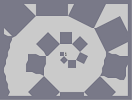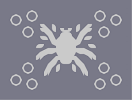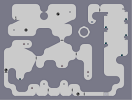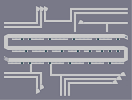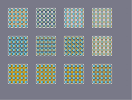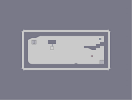Blocky Spiral 6-Chamber-Parasite No Exeptions Supervision Experiment: Green N Chronicles part01

### At first, I didn't understand

until I saw how awesome the dagger was.

### Owch.

poor card.
Wait a sec - Is that an Ace-of-Hearts?

### sick

very cool and symbolic :)

### It's pretty good,

Obviously not as good as some of your other n-arts.I'll give it a 4. Better than anything I can do.

### hmm...

is that a dragonforce reference i see?

### yup^^

love it its AMAZING!

### O M G

That is F***ING AWESOME!

### O M G

That is F***ING AWESOME!

### cool

i dont understand it but its cool

### KEWL

ALL OF UR N ART ARE AMAZING
AWESOME, 5AVED

### CHERIO!

You plonker you.
just killn, looks uber.

### me858

you're not supposed to play it, it's art. To dragon, very nice. 4/5

### me858

you're not supposed to play it, it's art. To dragon, very nice. 4/5

### how

how do i play this map????

### hmm

i think thats not possible with this map ^^'

too much objekts. And the rocketturets will make it lag and kill the player instantly...

why not
but try if u want

### I love it

5aved

Can I download ur map and make it a playable map? Would you care?

Can I download ur map and make it a playable map? Would you care?

ur back!!!

### hmmm

I don't really like it.. and for me - it should not be bitesized.. just too simple.. and messy...

Your dragons are very cool, this one... I just don't like it.
N/R

### Cards a bit thick

but that cant be helped so still 5/5

### Wait, no.

Disregard the Harry Potter thing.

### Harry Potter?

Nice.

is awesome! It brings realism to the image. It's supposed to be a shadow right?

### no

you never said that! then I am still anxious, thanx alot
hehe :P

### ^^

@kkstronger0

@bunniesandsheep
i remind to wrote, that i would submit a map from time to time... please keep that in mind. I still have not much time ^^'

@99turtle99
thx. I thougt of that Z-Twump blue + gold too. But that wont work on my PC. Its not fast enough for that ^^
And that drone-gold-green looks a little bit like a grid.
but anyway thx for the idea. ^^

BITESIZED!
wooooo yaaaa!

### Looks cool.

The lines in the card deck aren't really straight when looking in full view, but it doesn't jump out from the thumbnail. Some other minor flaws here and there, but it's still nice work.

### yay!!!!!

dragon's making n-arts again. rise up against mrgy!!!!

### well

http://numa.notdot.net/map/91108

if you're looking for green, that may help.

### SOOO AWESOME.

No wonder Dragon_Moon made it! ;)

5aved

http://numa.notdot.net/map/90867

5/5

### Great!

Continue making maps! Your maps are totally my favorite N-Arts.

5/5

### O MY GOD THAT'S

AWESOME!!!!!!!!!!!!!!!!! 5/5 FROM ME + FAVED!!!!!!!!!!!!!

### 5/5

i love you. and you started making maps again!

i love it

Great job.